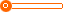# 汽车座套激光裁剪机下料机

1 台

(发货期限：自买家付款之日起 天内发货)

• VIP指数:[第1年]
• 认证信息:
• 所在地区：山东
•• 详细说明
• 规格参数
• 联系方式
r

r AOL-1610汽车用品 r

r

r 激光切割机   r

r

r r

r

r   r

r

r   r

r

r 这台设备可以裁汽车真皮座椅、汽车座套、坐垫、避光垫等汽车内饰产品。
r
这台设备可以为您节省请打板师傅的高额工资
r
这台设备可以为您节省百分之20的材料
r
这台设备可以为您提高一倍的工作效率
r
这台设备可以让您自己创业致富
r
奥镭激光 是您致富的好帮手！现在买机器 赠送各种电子版图。
r

r
r

r

r 【机型特点】 r

r

r     敞开式设计，加工高效、效果好，精度高，尤其适合大幅面连续线条雕花，各种材料座套的切型、下料。采用履带式工作平台，可配合送料装置（选配），对座套材料进行快速连续切割。切割系统可对排版后，单个排版长度超过切割幅面的超长排版，进行整版完成裁剪。可选配智能排料软件，对切割图形进行快速省料排版。 r

r

r 【适合材料】   r

r

r     无纺、丙纶、混纺、真皮、仿皮、复合人造革各种座套材料。 r

r

r 【适用行业】     r

r

r 适用于汽车座垫，座套，各种幅面地毯，门前毯，汽车飞毯，长毛毯等切割、雕花、拼花。 r

r

r 【强大的软件支持】 r

r

r 1、连续拼接功能，一版内无法完整切割的图形会自动延迟到下一版完整切割，图形内部小件切割与外框在同一次送料中切割，辅助智能排版系统能最大程度有效合理利用材料。 r

r

r 2、自动识别功能，软件可自动识别中文标记不切割只做电脑显示。 r

r

r 3、断电续切功能，断电后设备能自动记忆切割状态，通电后可从同处继续切割。 r

r

r 【设备优势】
r
1、良好的光学系统，激光功率稳定，寿命长
r
2、每分钟可高达500个激光穿孔，满足高效生产的需求
r
3、高精度步进马达驱动，高级精密同步皮带和带轮传动，保证高速的运动和良好的运动精度
r
4、具有废料收集箱，清理切割废料更加便利
r
5、采用先进的DSP控制技术，能实现快速连续曲线切割
r
6、加工范围广，自动化程度高，全电脑控制
r
7、精度高、速度快、性能稳定、操作简单、维护方便
r
【技术参数】 r

r

r   r

r r r r r

r 快速曲线切割功能 r

r r r

r 任意曲线均能快速连续切割 r

r r r r r

r 支持格式 r

r r r

r r r r r

r 多文档功能 r

r r r

r 可存储多达128个作业文件，在完全脱离计算机的情况下，用户可以在雕刻机上进行选择文件，轻松实现128种不同产品的批量生产 r

r r r r r

r 用于行业 r

r r r

r 专业适用于汽车用品雕花切割、服装皮革雕花、切割、镂空、商标切割、毛绒玩具切割成型、模型制作行业等 r

r r r r r

r 加工面积 r

r r r

r 1600x1000mm 1800x1200mm 1600x2000mm 1800x2500mm r

r r r r r

r 激光类型 r

r r r

r 玻璃内腔式封离CO2激光器（符合GB11748-89标准）（可选配射频激励式金属管）波长10.6um r

r r r r r

r 激光功率 r

r r r

r 80-150W（可选） r

r r r r r

r 激光管冷却 r

r r r

r 纯净循环水冷却 r

r r r r r

r 能量调节 r

r r r

r 0-100%无段控制、软件内部0-100%可调 r

r r r r r

r 控制及驱动 r

r r r

r 高速DSP控制、步进电机高细分驱动 r

r r r r r

r 雕刻速度 r

r r r

r 0-1000mm/s r

r r r r r

r 切割速度 r

r r r

r 0-400mm/s r

r r r r r

r 工作电压 r

r r r

r 220v 50HZ r

r r r r r

r 定位精度 r

r r r

r ≤±0.01mm r

r r r r r

r 整机功率 r

r r r

r ≤1500W r

r r r r r

r 工作环境 r

r r r

r 温度：0-45  湿度5%-95%（无凝水） r

r r r r r

r 软件支持格式 r

r r r

r r r r r

r r

r

r r

r

r r

r

r   售后服务 r

r

r   r

r

r 1、售前为客户提供雕刻机基本知识及使用咨询服务，推荐最适当的机型。 r

r

r   r

r

r 2、售前免费为客户打样，从技术上协助客户完成加工制作工艺。 r

r

r   r

r

r 3、售前免费进行使用培训，客户可学会后再购机，以减低投资风险。 r

r

r   r

r

r 4、上门维护维修处理时间不超过24小时，保修期内免费维修。 r

r

r   r

r

r 5、对于本地的客户，如果雕刻机出现故障而未能及时修复，客 r

r

r   r

r

r 　 户有加工订单急需交货的，可到我公司免费使用雕刻机。 r

r

r   r

r

r 6、经常上门回访，解决可能存在的问题和隐患，并协助客户提高 r

r

r   r

r

r 　 使用技术，交流使用经验。 r

r

r   r

r

r 7、客户使用中遇到疑难问题可随时咨询。 r

r

r   r

r

r 8、免费为客户提供常规雕刻材料、雕刻等供应商。 r

r

r   r

r

r 济南耀邦数控设备有限公司
r
地址：山东济南历城区工业北路159-32 r

r

r 联系人：赵然 r

r

r QQ2874314368 r

r

r 网址： r

r"};

查看更多同类代理产品
上一组 下一组

## 您可以通过以下类目找到类似信息：

免责声明：以上所展示的信息由会员自行提供，内容的真实性、准确性和合法性由发布会员负责。汽车大视野对此不承担任何责任。

友情提醒：为规避购买风险，建议您在购买相关产品前务必确认供应商资质及产品质量！